# Determining the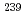Np(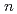,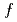) cross section using the surrogate ratio method
Neutron-induced fission cross section data are needed in various fields of applied and basic nuclear science. However, cross sections of short-lived nuclei are difficult to measure directly due to experimental constraints. The first experimental determination of the neutron-induced fission cross section ofNp at non-thermal energies was performed. The Surrogate Ratio Method (SRM) was employed to indirectly deduce theNp(,) cross section. The surrogate reactions used were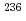U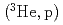and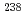Uwith the reference cross section given by the well-knownNp(,) cross section. The ratio of observed fission reactions resulting from the two formed compound nuclei,Np and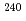Np, was multiplied by the directly-measured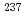Np(,) cross section to determine theNp(,) cross section. TheNp(,) cross section was determined with an uncertainty ranging between 3 - 30% over the energy range of 0.2 - 20 MeV.